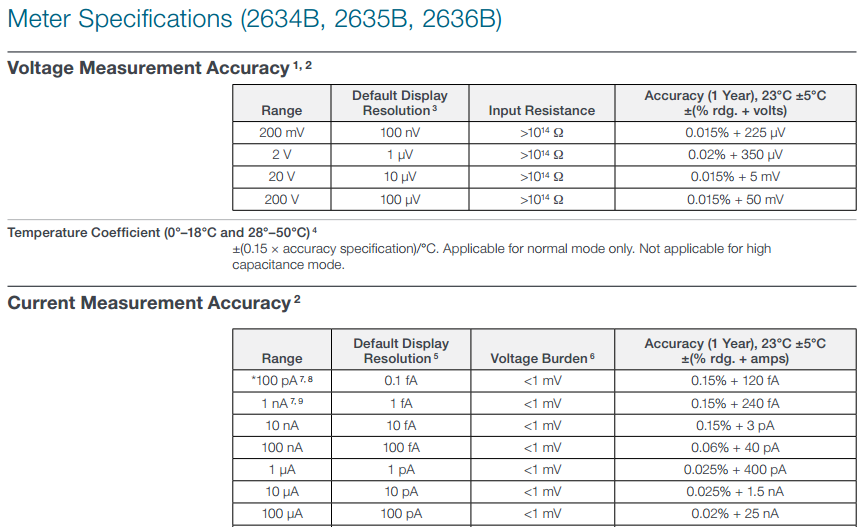# Keithley 2634B 数字源表SMU仪器（200V，10A，200W，2通道）

Voltage Source Specifications
Range;Programming;Accuracy (1 Year);Typical Noise (peak-peak)
Resolution  ±(% rdg. + volts)  0.1Hz–10Hz
200 mV 5 µV   0.02% + 375 µV  20 µV
2 V    50 µV  0.02% + 600 µV  50 µV
20 V   500 µV 0.02% + 5 mV    300 µV
200 V  5 mV   0.02% + 50 mV   2mV
Current Source Specifications
Range;Programming;Accuracy (1 Year);Typical Noise (peak-peak)
Resolution  ±(% rdg. + volts)  0.1Hz–10Hz
1 nA    2 pA    0.15% + 2 pA     800 fA
10nA    20 pA   0.15% + 5 pA     2 pA
100nA   200 pA  0.06% + 50 pA    5 pA
1µA     20pA    0.03% + 700 pA   25 pA
10µA    200pA   0.03% + 5 nA     60 pA
100 µA  2 nA    0.03% + 60 nA    3 nA
1 mA    20 nA   0.03% + 300 nA   6 nA
10 mA   200 nA  0.03% + 6 µA     200 nA
100 mA  2 µA    0.03% + 30 µA    600 nA
1 A     20 µA   0.05% + 1.8 mA   70 µA
1.5A    50 µA   0.06% + 4 mA     150 µA
10 A    200 µA  0.5% + 40 mA (typical)
Transient Response Time <70 s="" for="" the="" output="" to="" recover="" to="" within="" 0="" 1="" for="" a="" 10="" to="" 90="" step="" change="" in="" load="" br=""> Voltage Source Output Settling Time
Time required to reach within 0.1% of final value after source level command is processed on a fixed range.
Range Settling Time
200 mV <50 s="" typical="" br=""> 2 V <50 s="" typical="" br=""> 20 V <110 s="" typical="" br=""> 200 V <700 s="" typical="" br=""> DC Floating Voltage Output can be floated up to ±250 VDC from chassis ground.
Remote Sense Operating Range 1 Maximum voltage between HI and SENSE HI = 3 V.
Maximum voltage between LO and SENSE LO = 3 V.
200 V Range Max. output voltage = 202.3 V – total voltage drop across source leads (maximum 1 Ω per source lead).
20 V Range Max. output voltage = 23.3 V – total voltage drop across source leads (maximum 1 Ω per source lead).
Over Temperature Protection Internally sensed temperature overload puts unit in standby mode.
Voltage Source Range Change Overshoot
<300 mv="" 0="" 1="" of="" larger="" range="" typical="" overshoot="" into="" a="" 200="" k="" load="" 20="" mhz="" bw="" br=""> Current Source Range Change Overshoot
<5% of larger range + 300 mV/Rload (typical with source settling set to SETTLE_SMOOTH_100NA). See
Current Source Output Settling Time for additional test conditions
With handle and bumpers: 89 mm × 213 mm × 460 mm
With bumpers and handle: 5.5 kg

Details